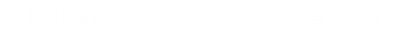Skip to main content# Collaborati: Settings > Discounts in LEDES

This is a reference article describing discounts in LEDES files for Collaborati.

Regarding the Setting “Discounts in LEDES” in Collaborati, if this setting is set to "derived from a percentage"
then your client's system would recognize that the discount is a percentage.

If there was an adjustment made in your client's TeamConnect system, then that would still recognize the discount as a percentage, rather than a flat amount.

Previously, if the adjustment was made in your client's system, the discount would only be recognized as a flat amount.

Here is a simple example.

• One fee entry: Rate = 100 and Hours = 10  with a percentage discount of 10% .
• Line item/Invoice total = 1000 - 100 = 900 .

In your client's system, your client might discount the rate from 100, to 80.

• This adjustment would include the percentage discount.
• Adjusted Rate = 80 and Hours = 10 and percentage discount of 10% .
• Adjusted Line item/Invoice total = 800 - 80 = 720 .

*** Previously the discount would have only recognized the flat amount:
Adjusted Line item/Invoice total = 800 - 100 = 700 .

• Was this article helpful?